# A New Approach to the Theory of Everything (VMTE)

Nimit Theeraleekul B. Eng. (Communication)

(17 March 2004, 3 rd. revised July 1, 2011)

Abstract: Modern theoretical physicists have been struggle for a long time for finding the theory of everything. Nowadays, it is well-known that the obstacle was the incomplete and inconsistent of the theories using as tools; especially the two main theories, general theory of relativity and quantum mechanical theory. Modification based on new concept of Vacuum Mechanics will complete the tools, making them consistent to each other, and then opening the gate to the desired theory.

# Content:1) Introduction. 2) Something may be wrong with modern theories in physics.2.1) Problems with Maxwell electromagnetic field theory.2.2) Problems with Einstein special theory of relativity.2.3) Problems with Einstein general theory of relativity.2.4) Problems with quantum mechanical theory.2.5) Inconsistency between GTR and QMT. 3) Vacuum mechanics -- a new conceptual mechanics for all natural phenomena. 3.1)Vacuum medium is the fabric structure of vacuum space.3.2)Electrons are tiny black holes 3.3) Origin of matters. 3.4) Mechanism of gravity. 4) Improving MaxwellÆs theory of electromagnetic field. 5) Improving EinsteinÆs theory of special relativity. 6) Improving EinsteinÆs general theory of relativity. 7) Improving quantum mechanical theory. 8) Relativity and quantum theory could be married now!9) Appearance of the theory of everything.10) Conclusion & discussion. 11) References.

1) Introduction. Theory of Everything (TOE) was defined as one which was aimed to unify all the four basic forces of nature, i.e. gravity, electromagnetic, weak and strong forces . And modern theoretical physicists have been struggling to find it for a long time, but there was no sign of successfulness until now!

Anyway, string theory seems to get more attention as the TOE, but it has one serious problem, i.e. it is involved with extra dimensions which is not so realistic! Another proposed theory is quantum gravity, but its main problem is the incompleteness and the inconsistency of the theories involved, i.e. general theory of relativity (GTR) and quantum mechanical theory (QMT) .

For me (the author), I am not so happy with any extra-dimension theory. Why nature has to hide the extra dimensions? There should be a simple intuitive way for her to build the universe and here, this article ōVacuum mechanics: A new approach to the theory of everythingö is one that we asked for! And also we will see later that even EinsteinÆs dream via a five-dimensional theory, could be done with our familiar four-dimension. In VMTE, it is no need for the fifth dimension. Instead, we just used what which was called ōcondensed of vacuum mediumö or something like a tiny black hole to do the job!

2) Something may be wrong with modern theories in physics. Nowadays, we know that the two great modern physics theories are GTR and QMT. While GTR is responsible for physics in macro scale up to the universe, in contrary to QMT which is responsible for physics in micro scale down to atoms and elementary particles. Despites their successful in accuracy and predictability; both of them are incomplete and inconsistent to each other. Here we will show what the main problems of GTR and QMT are. And because special theory of relativity (STR) is the basic foundation for GTR, so we should reconsider it first. Anyway we also know that STR was invented to solve the problem in electrodynamics. Then it is inevitable to find out the problem of MaxwellÆs electromagnetic field theory (MET) in which electrodynamics was based on first!

2.1) Problems with Maxwell electromagnetic field theory. Historically, when James Clerk Maxwell developed the theory, he created a mechanical model called a granular model of space. In this model, aether medium was used as the mechanism for electromagnetic field . But today most of physicists do not believe in the model, i.e. the idler wheels and gears of the aether. Only the equations were accepted and still being used. For the author opinion, this is the first sign of the troubles that will follow then.

Reference to the concept of Maxwell model in which the (luminiferous) aether was the medium filled in vacuum space and responsible for propagation of electromagnetic waves. This is the same concept as all kind of waves in the nature do. But when electrodynamics was born, electromagnetic field theory did not work in the ream of classical mechanics. To solve this problem Einstein had proposed special theory of relativity, and which was accepted. And at same time the theory had rule out the existence of the aether, so this is the second sign of the problems that will occur afterward!

If the aether was abandoned from being the elastic medium filled vacuum space, then what is the mechanism of radiation process  and how do electromagnetic waves propagate through the vacuum space? Most of all standard textbooks have the difficulty for clearly explanation; they only said that the wave is self-contained oscillations that propagate by mutual creation between the changing of electric and magnetic field. But that will violate the principle of causality, because both of the changing of electric and magnetic field is simultaneous created by the changing of electric current supplied by the power source .

Some one may ask that, is the author still believes in the aether theory? How about the long debated about the existing of aether during the nineteenth century, in which the negative result of the expected interference from the famous Michelson-Morley experiment has ruled out the existence of the aether? We will talk about the second question latter, for the first question the answer is yes and no. Yes, the author still believe in general concept of ōsomethingö like the old aether, but not the aether itself. And no, because it not something that filled the vacuum space like the aether, but it is something more special -- it is ōvacuum medium spaceö itself. And we will see its detail next.

2.2) Problems with Einstein special theory of relativity.  Everyone who familiar with STR would found that its main philosophic problems are the increasing mass, length contraction and time dilation of the moving object. What are the causes of these effects? No one could understand and can explain clearly why it is something like that! Beside the problems mentioned, the second hypothesis (of the theory) ōthe velocity of light is the same for any inertial observersö is also criticized to be incorrect.

Now, if there is something which is physically acts as the ōcauseö in the mentioned problems, then we may know the ōeffectö. Indeed, latter we would found that something which we ask for, it is vacuum medium (instead of empty vacuum space). And we will see that it is easily to solve the mentioned problems by using the mechanism of the vacuum medium!

2.3) Problems with Einstein general theory of relativity. Because GTR was extended from STR by including gravity, so it has the same philosophic problems as STR do. Further more GTR itself was born with another serious philosophic problem about space-time. The theory says that space-time could be curved and distorted by the gravity, it is alright. But remember that is vacuum space is empty, then how space without ōanythingö could be curved? What is it mechanism? Thing get worse when the theory say that gravity wave could propagate by the vibration of empty space-time. This is crazy, and how could we understand it?

Nowadays modern cosmologists have found that our universe is expanding! And according to the calculation using the conventional (non-ether based) GTR, they found that dark energy is needed to give enough mass density for the acceleration of the expanding. Again, if there is something which is a physical medium involved in the vacuum space, then the problems may be eliminated. Indeed, later we would found that something which we ask for; it is vacuum medium space (instead of empty vacuum space).

2.4) Problems with quantum mechanical theory. Let us start with an outstanding Nobel Prize laureate in physics, Richard P. FeynmanÆs word when talking about quantum mechanics. He said that ōI think I can safely say that nobody understand quantum mechanicsö . And he went further (when talking about electron waveÆs behavior which appeared as the interfering pattern after moving through double-slit experiment.) ōģ how does it really work? What machinery is actually producing this thing? Nobody knows any machinery. ģö [6Æ]. Does it mean that quantum mechanics has no mechanism? Of course not, quantum mechanics is science, not a magic! So the central problem is about atomic particle wave, not only because no one knows what the particle wave is, but also no one knows what its mechanism is.

Fortunately, we know that the most essential part of every kind of wave in the nature is the requirement for a physical medium to be the carrier of the wave! Also we know that in QMT, the moving electrons in the experiment moving through ōnothingö in vacuum space. This is crazy, how could electron wave was created without any carrier for the wave? But what will happen if the vacuum space is vacuum medium space? The answer is that it is now possible for the moving electrons to create wave while they are moving!

2.5) Inconsistency between GTR and QMT. It is interesting to note that we use both theories to explain natural phenomena in the same of our single world (within our single universe), but they contradict to each other. While GTR was responsible for the macro world i.e. from our solar system up to the whole universe; QMT was responsible for the micro world i.e. from molecule, atom down to electron. And while QMT say that there are infinite energy in empty vacuum space, GTR say that there is nothing in there! Or while GTR say that energy come in continuous value, QMT say that energy is quantized. Then the question is why both the two great theories say different thing, and which theory is right?

Up to now, from all of the above problems we could get some clue that it is because we have ōnothingö to deal with. But if we have vacuum medium which acts as the mechanism of every phenomenon that occurs in EMT, STR, GTR and QMT, then may be we could solve the mentioned problems! Now, what is vacuum medium and how to deal with it? To answer the question we have to be done in three steps. First, making an assumption of the vacuum space medium and proving it. Second, creating a suitable model and applying to the real world. Finally, using it for solving the problems and seeing whether it is work or not. So let us start from the first step; a new conceptual hypothesis.

3) Vacuum mechanics -- a new conceptual mechanism for all natural phenomena. In physics, when we deal with rigid solids, we use the mechanics of rigid bodies as the tool. And when dealing with liquids or gases what we used is fluid mechanics. In the same way, we should use vacuum mechanics i.e. the mechanism of vacuum medium when dealing with vacuum medium. But what is vacuum medium and how it relates to all the existing matters? How this vacuum mechanics could cover the mechanism of all natural phenomena? Before answering, first we will start with detail of vacuum medium, and then at the end of this section we will found why and how it is be so!

3.1) Vacuum medium is the fabric structure of vacuum space. This is the fundamental hypothesis. Or we could equally say that vacuum space was created using vacuum medium as the raw material. So there is no such thing which was called as the conventional empty vacuum space (without anything), or there is not something like the old familiar aether that filled the empty vacuum space. Instead there is only vacuum space that coexists with vacuum medium, and we will call it as vacuum medium space!

Now what really is vacuum medium? Vacuum medium is the geometrical structural contraction potential energy; the energy that each of its infinitesimal part holds each other together by its internal contraction force and forming to be the physical fabric structure of our space. Note if we interpret that the existing of vacuum medium as positive energy and its internal contraction force as negative energy, then the total energy is zero. So the principle of conservation of energy is preserved!

Next what is the contraction potential energy (of vacuum medium)? Traditionally, energy was usually not to be regarded as substance; it is abstraction and by definition it is the ability to do work. But here, based on the ontological concept , vacuum medium (energy) is a new kind of substance! It is an entity of a physical reality. And the most important point is that this vacuum medium is the only one primordial substance; the substance that forming to be everything in our universe. In other word we may say that the universe is nothing but vacuum medium and its products!

Actually vacuum medium energy is the same thing as what which we called ōgravitational field energyö that was created by ordinary material mass (near by) that we are familiar. But for vacuum medium, its gravity is aroused within itself from its own mass (its internal contraction force). So it acts as the background energy of vacuum medium space, and it is the lowest energy level in the universe (the energy that just enough to form the structure of empty universe excluding ordinary matter)!

[Note that when an ordinary material mass was placed in vacuum medium space, it will create the conventional gravitational field around. But the question is what this conventional gravitational field is and how it was created? The answer is it is the internal stress in vacuum medium which was created by the contraction force of the material mass nearby (because the mass, which we will see latter, is the condensed of vacuum medium).]

In more technical detail, vacuum medium is a continuous isotopic homogenous medium which have a peculiar mechanical property. It is very thin in mass density so it is permeable by all matters almost without any observed resistance! But it has very large elastic coefficient and sensitive to shear force (rotational force) while not to compressive or longitudinal force. The reason is because normally the elastic resistance against change of volume is larger than that change of relative orientation , especially if the volume is the fabric structure of the whole space of the universe!

But how much is the mass density of this vacuum medium? Of course we could get the answer according to mass ¢ energy conversion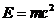if we know the energy density. Another possible way is to calculate from the dynamics of vacuum medium itself and which it was shown in CGTR . And there we have found that the mass density of vacuum medium energy is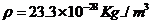which is very thin indeed!

By the way, to imagine how the vacuum medium look likes, it is instructive to compare it with our breathing air. What similar between air and vacuum medium is that both of them are transparent. While it seems that airÆs mass density is very dilute (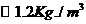), but we could see from above paragraph that vacuum medium mass density is much more dilute. This is the reason why it is very difficult to detect its existence directly.

Anyway, there are two important different points between air and vacuum medium. First, air construction is composed of billions of its molecules which are discrete values, while vacuum medium is an ideal isotopic continuous medium. Second, vacuum medium has a very large elastic coefficient and sensitive to shear force or rotational force, while air is sensitive to compressive or longitudinal force. This is the reason why sound wave in air is a longitudinal wave, while light wave in vacuum medium is a shear wave!

Finally, we often heard that vacuum medium should be very rigid for creating such a huge speed c of light! This idea comes from the concept that sound speed in rigid medium such as steel which is greater than the non-rigid medium such as air, for example. But this is not quite right; the better method is to compare the same kind of medium such as normal air and hydrogen. For airÆs mass density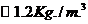which has sound speed 331m/s, while hydrogen mass density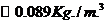has sound speed 1284m/s. The reason is because mass density of hydrogen is lighter than the air (assumed both have the same modulus), so its sound speed is higher than air according to wave speed formula which equal to square root of modulus over density. And because vacuum mediumÆs density is much more dilute than air, so it is not surprise that light wave speed in vacuum medium must higher than air sound wave speed!

Experimental verification. To verify the hypothesis, the crucial point is to prove that vacuum space is not just an empty space without anything; instead it is equipped with vacuum medium. Two indirect experiments for proving that there is something physically existed in vacuum space (i.e. vacuum medium) will be discussed as follow.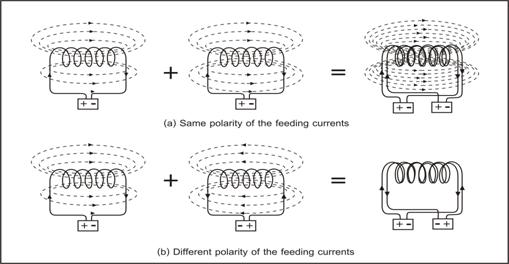Figure 1. Two¢solenoid experiment.

The first experiment is a simple one; it is a two-solenoid experiment as show in the diagram figure1. The concept used in this experiment is an elementary physics; it is about the magnetic field B generated by a direct current flowing in a solenoid according to the formula,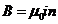ģ (1). [Where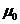is the permeability constant,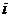is the feeding current and n in number of turns.]

When both two nearly identical solenoids are feed with the same amount (and same polarity) of direct currents as in (a) then the sum of the generated magnetic field is double. But when the feeding currents are opposite as in (b) then the sum of the generated magnetic field is zero! Someone may say that it is what it should be, yes it is, but where is the generated energy gone? It is not possible to be something like that, because we still feed the same amount of energy into both solenoids. Is this means that we can violate the law of conservation of energy? Of course not, we can not do something like that! The only one sensible explanation is that there is a cancellation of the opposite phase of internal rotational stress in the vacuum medium. So this means that vacuum medium is existed, doesnÆt it?

Anyway, to make it easy to visualize how the input energy was used for the cancellation of the opposite phase (of internal rotational stress) in the vacuum medium work. Let us look at a simpler analogy experiment which was used to demonstrate the interference of sound waves as show in the diagram figure 2. The experiment was done by feeding sound waves from loudspeaker into the tube and dividing into two equal branches, and then they combine again before going out at the output.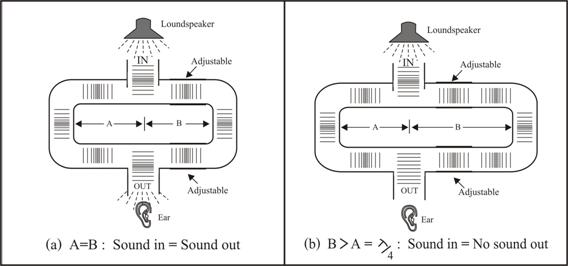Figure 2. Acoustic interference experiment.

If the length of the two branches is equal, then what we got at the output is the same level of sound waves as the input. But if the two branchesÆ length is different with a halve wavelength of the sound wave, then ideally, the sound level at the output is zero due to the destructive interference of the waves from the two branches.(For the real experiment we still got some very low level due to imperfect of the equipment.) Also we could interpret that this experiment demonstrates how the input energy was used to cancel internal stress which occurred in air medium!

The second experiment is a more practical one; it is involved electromagnetic field energy of VHF radio system. It is a normal way for sending VHF radio signal to a desired direction. The system consists of a two-element directional antenna as shown as in figure 3. Two half-wave dipole antennas are placing side by side with spacing of one fourth of the radio wavelength apart To operate the antenna system, two sinusoidal electromagnetic signal currents with opposite phase are fed into each of the antennas simultaneously. Then the total power density pattern of the radiated radio waves (calculated from the formula in 3 (b)) was created as shown in diagram 3 (a). , .

[Note, actually the total power density pattern is 3-Dimesion but only 2-Dimension is shown. It is an apple shape pattern in which the concave part (right hand side) point to the desired direction]

Next let us do the experiment, if both antennas are moved close together while the feeding currents keep fixed. The power density pattern will be decreased, the more closing of the antennas the more decreasing of power density pattern in (b) (n=1/4 is biggest, n=1/8 is smaller and n=1/12 is smallest). According to the theory and the formula, when both antennas are at the same position, the size of the power pattern will reduce to zero. Now the question is where is the radiation energy of the radio wave gone? Someone may say that it is obviously a simple destructive interference phenomenon of waves. Yes, it is, but that will violate the law of conservation of energy. (We have kept the same input energy but the output energy is decreased.)  The only possible explanation to the question is that; there is the cancellation of the opposite phase in the internal stress of vacuum medium.

By the way, we could also make it easy to visualize by using an experiment analogous to the acoustic wave in fig. 2, but we will use microwaves instead of acoustic wave. Here the equipment is a square metal tube which was called ōwave guideö together with microwave signal generator and microwave signal receiver. Then what we will get is the demonstration of the interference of microwaves in the same way as what was got from the sound waves. And what interesting here is that it can be an indirect prove for the existence of vacuum medium!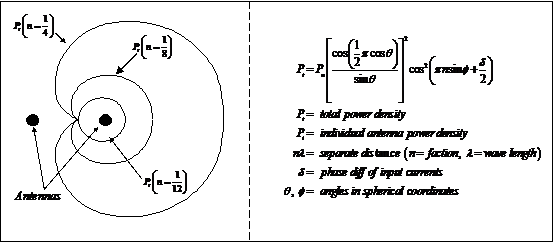Figure 3. Radio radiation patterns of a two half-wave antennas & the formula.

3.2) Electrons are tiny black holes. This is the consequent hypothesis. It was inspired from the concept ōmatter is the condensed of energyö and from EinsteinÆs electron theory . The first inspiration is quite obvious from the equivalence of mass and energy, and the important example is the action of an atomic bomb.

According to EinsteinÆs theory of electron, its concept is that material masses of electrical elementary particles (electron or proton) are held together by gravitational forces. Anyway, it faced with difficulty that ordinary gravitational force is too weak to do the work. But under our new concept of vacuum mechanics, electron is a tiny black hole which was formed by gravitational force of vacuum medium itself. (Unlike a conventional black hole which was formed by gravitational force of the collapsed of huge matters.) So it needs not to overcome huge Coulomb repulsive force of the matters.

In summary, Einstein approached the inquiry into the structure of the material particles based on the assumption of a material energy-momentum tensor in the present of gravitational fields together with MaxwellÆs energy tensor. Finally, he had got the desired formula (2) below.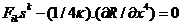ģ. (2).

The first term in equation (2) is the Coulomb repulsive force which is held in equilibrium by a gravitational force in the second term. Of course, this seems impossible because Coulomb repulsive force is much stronger than gravitational force. Anyway, based on our new concept that electron and proton are tiny black holes, we would found that the problem was easily eliminated!

Now by using the Reissner-Nordstrom solution, equation (3), together with the concept that electron is a tiny black hole immerged in the surrounding of vacuum medium, then we would found that Reissner-Nordstrom solution should be a rational model of electron which could staying stable. (Actually (3) is the solution of Einstein-Maxwell equation, which is the spherically symmetric, static, exterior field of a charged particle. Originally, it was used for considering the gravitation field of a charged particle and was hoped to be a useful model of electron, but failed!)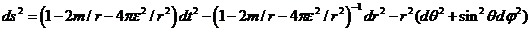ģ. (3).

[Where the second term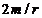in parenthesis is the gravitation field due to electron mass, and the third term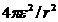is the gravitation field due to electron electric field.]

According to diagram (a), it shows an electron sphere with radius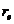surrounding by vacuum medium. On the surface of the sphere, the suction force of electron black hole was countered by the internal tensile force (stress) in vacuum medium around, so the total force on the surface of the sphere is zero! In this situation theterm must equal to theterm, or equivalently we may say that the effect of curved space due to gravity was canceled by the effect due to electric energy. And then the line element metric will be Minkowski (flat) space-time there!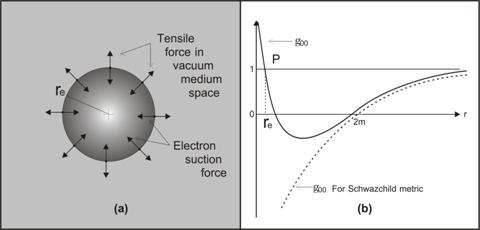Figure 4. Electron black hole model.

Now according to diagram (b) which is the graph between the metric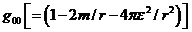of Reissner-Nordstrom equation, and the radius r , we would found that at point P where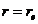,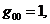and we could calculate that electron radiusis equal to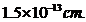Indeed, we also found that it is the same order as the classical electron radius, so it give us confidence in the proposed electron black hole model!

(Someone may wonder why the black hole does not suck the surrounding vacuum medium. Yes it does, by creating the internal stress in the surrounding vacuum medium. But the tiny black hole can not suck the entire vacuum medium into it. Because to do so, it has to suck the entire universe! (Remember that vacuum medium is the fabric structure of vacuum space.)

Theoretical verification. Now we will do an indirect prove the hypothesis by using it to solve the unsolved problems with the energy and mass of an electron (a point charge particle). And here we will use the method analogous to what which used by Richard P. Feynman  as follow.

First we will calculate field energy of an electron. It was supposed that electron has a simple model, in which all of its charge q is uniformly distributed on the surface of a sphere of radius a.  Now letÆs calculate the charge energy from its electromagnetic field. When the electron is standing still, the energy per unit volume is proportional to square of electric field. That is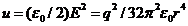To get the total energy, we integrate the energy density over all space. Using the volume element 4¶r² dr, while the lower limit is a, and the upper limit is ∞. Then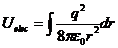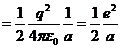ģ.  .(4)              [where e² =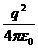]

From equation (4), the calculated electrostatic energy is the energy of the internal stress in the surrounding vacuum medium which was created by the suction of the existing tiny black hole. So the ōself energyö of electron (the black hole) is also (½) e²/a, this because the energy created from a source = energy of the source. Then the correct (total) energy of the electron is as (5),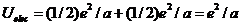ģ.. (5),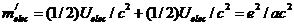ģ.. (6).

And electron mass is the sum of the mass due to the self energy (mechanical mass) plus the mass due to the created energy (electromagnetic field mass) as in (6)!

Second, to calculate the field momentum of an electron, we suppose the sphere of electron (mentioned above) has no mechanical mass. It is moving at a low uniform velocity v compared with the speed of light. Then we could found the momentum of the electron is as (7)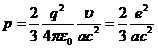ģģģģ (7),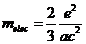ģģģ  (8).

Because the momentum of the field (electromagnetic momentum) is proportional to v, so the coefficient of v is electromagnetic massof the electron, is as (8).  But if we include the self energy of electron (½) e²/a, then the total momentum of the moving electron is twice of (7), and electron mass is also twice of (8). That is as shown in (9).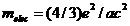ģģ (9),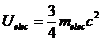ģģ. (10).

Now we can see thatin (6) is not the same as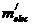in (4)! The problem gets worst when we combine equations (4) and (9) together, and what we got is as in (10). And it is clear that (10) violates the equivalence principle of energy and mass (U =mc²)! What is wrong? No one knows until now. But here we will solve the problem by using the concept that electron is a tiny black hole immerged in vacuum medium space below.

To solve the problem of the energy in (10), we will refer to the method for finding energy of the fluid which acts on the immerged moving sphere in ōHydrodynamicsö . And according to the method mentioned, the kinetic energy of the fluid acting on the sphere is equivalent to one forth of the mass of the fluid displaced. In our case the addition mass to the inertia mass of electron is one forth of the mass of the vacuum medium displaced which is also one forth of the mass of electron; that is (1/4)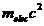, then the correct energy and energy - mass relation in (7) is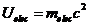ģ. (11) as predicted by the theory of relativity!

In conclusion, we have solved the problem in which the equivalence principle of energy and mass is preserved. And from (4) and (11) we could get one correct mass of electron with one correct of its energy that is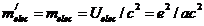. Then we could say that we have done (indirect) theoretical prove for the hypothesis!

3.3) Origin of matters. From the two previous sections, we have learned that vacuum medium is the fabric structure of vacuum space, while electron is the condensed vacuum medium. In the same way as electron, proton could also consider as another condensed vacuum medium but with a heavier size! Both of them are the only two elementary particles in the universe. Now we will learn how matter was created to be a part of our universe, i.e. vacuum medium universe!

Actually the new concept (that electrons and protons are tiny black holes) is more rational than the old conventional concept (electron is a negative charge and a proton is positive charge). For example, it is easy to explain electrostatic field and magnetic field phenomena.  When an electron or a proton are staying still, under this situation it will attach the surrounding vacuum medium. And this will create the internal stress in the vacuum medium around, which is appeared as electrostatic field. But when it is moving, it will rotate in the direction parallel to the motionÆs axis. So it will create rotational stress in the surrounding vacuum medium while dragging it along ¢ this is magnetostatic field!

And to give us some confidence about the new concept of charges particle are tiny black holes, we will use it to explain the interaction between charges particle. Conventional concept that ōdifferent type of charge particles attract while the same types repelö could be explained by the mechanism of vacuum medium. We know that ōany size (weight)ö of tiny black hole ōattractö each other. But because they also attract the surrounding vacuum medium, so under certain distance apart, the same sizes of tiny black hole repel each other, while different size attract. This is because under such condition the attraction force between the black holes was affected by the vacuum medium between. So the better concept is ōdifferent size of charge particles attract while the same size repelsö. (Please see detail explanation in CEMT .)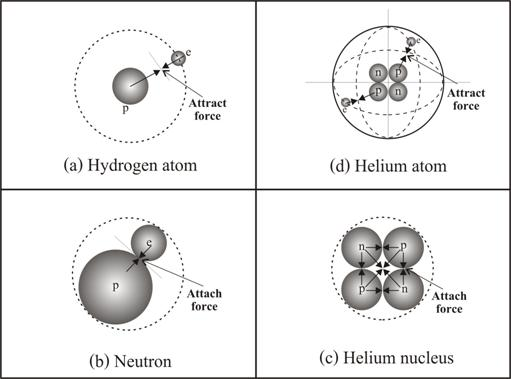Figure 5. Formation process of matters.

Now we will see how matter was created, when proton and electron come close together, they will attract each other and forming to be hydrogen atom as shown in diagram fig.5 (a). But they can not attach together because of the existing of vacuum medium between! Anyway, if they were forced to attach together and tearing off the vacuum medium between, then a neutron particle will be created as shown in (b). And the resultant is the ōattach forceö between different size particles (which was dominant after the compressing force had overcome the tensile force of vacuum medium) was named as ōweak forceö!

Next if two neutrons and two protons were forced to attach together, then a nucleus of helium atom will be created as shown in (c). And the resultant is another ōattach forceö between two same size particles (which was dominant after the compressing force had overcome the tensile force of vacuum medium), and was named as ōstrong forceö!

Finally, when the nucleus of helium atom was created, it will attract the near by electrons and forming to be helium atom. This is the initial state of the process for forming all kind of the matters in our universe by using two elementary particles i.e. proton and electron!

Up to now, we have seen what the vacuum medium is and its tiny black holes are. From here on we will find in more detail what the vacuum mechanics (i.e. the mechanism of vacuum medium) is, and how it behaves as the mechanism for all natural phenomena!  And first we will use it to improve the conventional EMT which has no working mechanism as point out early.

3.4) Mechanism of gravity. Under the concept that matter was composed with large amount of ōcondensed vacuum mediumö i.e. tiny black holes, then this is the reason that matters could attract each other. And this attraction force used vacuum medium as the transmitting medium (represent by contraction spring), and this is the mechanism of gravity as shown in the diagram fig.6 (b). In contrast to the conventional concept of gravity in the diagram fig.6 (a), we would found that we could not understand why and how gravity works!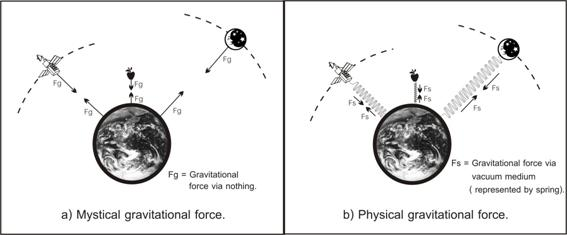Figure 6. Attraction mechanism between two masses.

4) Improving Maxwell electromagnetic field theory. Now, we have two main ingredients which are involved in electromagnetic field, they are vacuum medium and electron. While electron, i.e. charge particle, acts as the source of electromagnetic field, vacuum medium is the mechanism for transporting the field energy. So we are ready to complete Maxwell electromagnetic field theory by adding an appropriate and understandable mechanical model to show how it works.

New model of Maxwell electromagnetic field theory. Armed with vacuum mechanics, here is a more rational model which was built and explaining in short summary. Please see detail explanation of this section in article CEMT .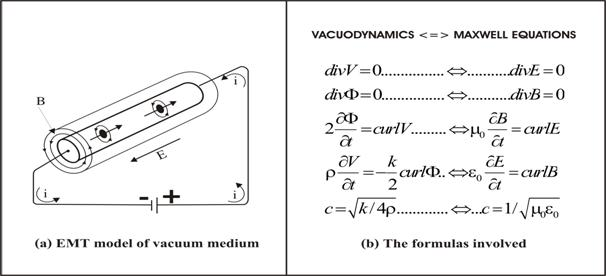Figure 7. Vacuum medium model of EMT and its derivation.

In the diagram 7 (a) shown, a simple direct current circuit is the proposed model. Stream of electrons (-i) was forced by potential difference to flow through the conductor with negligible resistance (for simplicity). Remember that electrons are tiny black holes, so while they are moving and rotating, they will drag the surrounding vacuum medium. The rotational stress in the vacuum medium which was created will be appeared as the surrounding magnetic field B. At the same instantaneous time the rotational stress in the vacuum medium will propagate along the wire and is appeared as electric field E!

To derive Maxwell equations from the new model of vacuum medium, we have used the guideline from the concept of ōthe quasi-elastic body model of aetherö developed in 19PPPPPPPPPPPthPPPPPPPPPPP century by Professor Arnold Summerfeld [8Æ]. This is possible because both of them have the same mechanical property; they are isotopic continuous elastic medium which is sensitive to rotation but not to compression!

By working out from stress-strain relations in vacuum medium, then what we got is the equations involved and having the same form as Maxwell equations as shown in diagram fig.6 (b). [Where for vacuum medium, V =velocity vector, F = angular vector, k = twist modulus and r = mass density, while for electrodynamics, E = electric vector, B = magnetic vector,= permeability and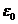= permittivity, j = current density (or density of the flowing tiny black holes of vacuum medium) and c = velocity of light.]

In conclusion, from the proposed mechanical model above we could derive the same Maxwell equations. So it should be an appropriate model for Maxwell electromagnetic field theory required! Also we could see that all electromagnetic phenomena are the appearances of the dynamics action of stress-strain relations in vacuum medium! It is a part of the mechanism of vacuum medium, i.e. vacuum mechanics, which we have called it as ōvacuodynamicsö!

Solution to the problems in Maxwell electromagnetic field theory. As an example, here we will show how to visualize the radiation process of electromagnetic wave as follow.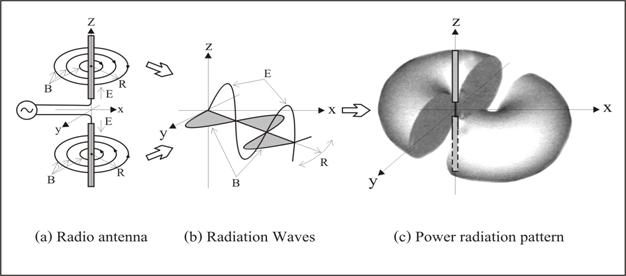Figure 8. Electromagnetic waves radiation process.

.

According to the diagram in fig. 8 (a), a half-wave dipole radio antenna is connected to a high frequency radio signal generator. The flowing up and down of electronÆs stream will create electromagnetic waves (of electric field and magnetic field) in vacuum medium around and then moving away from the antenna as shown in fig. 8 (b). When we calculate the total power of radiation field pattern, it is a donut shape as shown in fig. 8 (c).This is the radiation process of electromagnetic waves!

Finally it is easy to understand the radiation resistance from the radiation process above. In order to generate electromagnetic waves radiation, some input power must be used to oscillate vacuum medium which then create waves around the antenna. So the radiation resistance is due to the resistive action of vacuum medium. And to find the value of the radiation resistance of the antenna, we could do by calculated total power consumed in the circuit, and then divide it by the square of the flowing current. What we got is the radiation resistance for a half-wave dipole antenna and is equal to 73.26 ohms.

5) Improving Einstein special theory of relativity. Based on the concept of absolute vacuum medium reference frame, we have to reinterpret the conventional relativity principle because it valid only on the concept of empty vacuum space (which is existed only in the imagination). New interpretation is changing from ōPhysics is equivalent for any inertial reference frameö to be ōPhysics is equivalent in any frame reference to vacuum medium spaceö!  And what we have to do next is to derive the Lorentz transformation under the new concepts. (Please see detail explanation of this section in article CSTR .)

Derivation for the Lorentz transformation under the concept that vacuum medium space, which act as absolute privilege reference frame, is possible by using k-calculus as shown in the diagram fig.9. And we will see that this new derivation is simple and understandable, while for the conventional derivation it is difficult to do and can not understand why it is be so! Another advantage which we got via the new concept is ōPhysical meaningö of Lorentz transformation which could be understood and explainable i.e. it is the relative ōdistanceö and ōtimeö measured by using light speed c which propagated via vacuum medium (while Galilean transformation deal with imaginary infinite light speed via empty vacuum space)!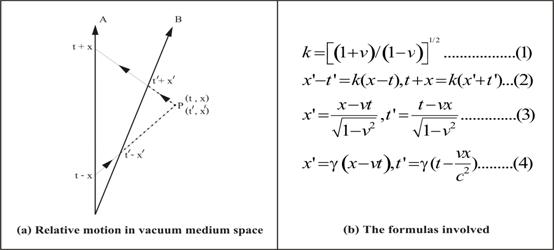Figure 9. Lorentz transformationsÆ derivation based on vacuum medium space.

Solution to the problems in Einstein special theory of relativity. First let us consider the moving mass which seems to ōincrease its intrinsic massö without any explanation why it is so! Instead if we accept that the mass is immerged in vacuum medium, then we could understand that the ōincreasing of massö represent the ōadditional inertial massö due to the ōinertial resistance of vacuum mediumö to the moving mass! This is analogous to the dragging force act on a moving car, a flying plane etc. due to the existing of the earth atmosphere.

Next, armed with the concept of vacuum medium space, it is easy to understand that relativistic concept for mass, momentum and kinetic energy, all is not the real increasing of their intrinsic quantity. It is just the effect of the dynamic resistance of vacuum medium in which they are invented to solve the unseen cause of unexplained experiment problems in relativistic dynamics. So we may say that ōrelativistic mechanics is the mechanics in vacuum medium space i.e. it is vacuum mechanicsö!

Finally we have shown that the conventional length contraction of a moving object is not a real value; it is a relative apparent length contraction which occurred as seen by a moving observer, so it should be called as ōrelative timeö! Anyway, for time dilation of a moving clock which should be a relative time, but because the moving clockÆs mechanism was slow down due to its increased mass, so it is a real effect of slowing time of the clock, but not a nature time dilation, so it should be called as ō relativistic timeö!

6) Improving Einstein general theory of relativity. Because GTR was extended from STR by including gravity, so we have to improve the base concept of GTR in the same way as the new concept used in STR, i.e. concept of vacuum medium space! So we have to reinterpret the conventional principle of equivalence because the principle of equivalence is valid only on the concept of empty vacuum space. So it has to change from ōPhysics is equivalent for any reference frameö to be ōPhysics is equivalent reference to vacuum medium spaceö! Please see detail of this section in article CGTR .

Vacuum medium universe ¢ a better view of our universe. Vacuum medium universe is the sea of vacuum medium as the main part; while all the existing matters i.e. galaxies, stars, dust and gas cloud etc. (condensed vacuum medium) is the minor part that immerge in the sea shown in the diagram fig. 6. And what we found in this new model of the universe is more rational and understandable than the conventional one. It could solve the problems in the conventional empty universe. Here is a short summary of some examples.

(Please be careful that what which was shown in the diagram is not true scale, it just showing how the overall view of the universe looks like for us who located at the center!)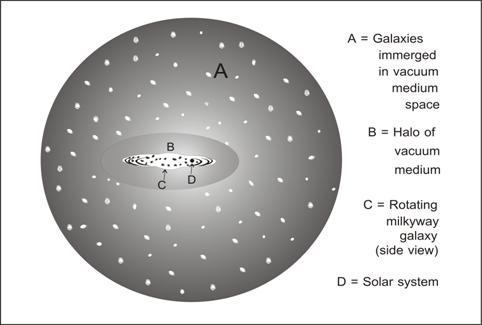Figure 10. Vacuum medium universe.

Dark energy problem. As mentioned early in section 2.3, it was found that our universe is expanding! And according to the calculation using the conventional (non-ether based) GTR, they found that dark energy is needed to give enough mass density for acceleration of the expanding of our universe. Based on the new idea of Vacuum medium universe we could see that actually the dark energy is vacuum medium which is the main part of the universeÆs total energy!

Someone may wonder why the tiny mass density of vacuum medium having so much accelerating pressure for expanding of the universe. The answer is because the universeÆs size is very, very huge (compare with galaxy as mentioned early in the diagram fig.6.), so the total mass of vacuum medium is also very, very large (compare with the total of galaxiesÆ mass and other observable mass). But on the contrary, for the scale of galaxyÆs size, the vacuum medium has a negligible effect on it.

Dark matter problem. Missing mass was found in all of galaxiesÆ abnormal rotation, i.e. their orbital rotational velocities do not obey Newton law of gravity (the velocity should decease while the distance from the center increases). Instead, the galaxies orbital rotational velocity is uniform for any distance from their center.

According to the stress action in very large elastic coefficient of vacuum medium, galaxyÆs rotation creates a huge stress energy which is then makes the galaxyÆs abnormal orbital rotational velocity, as if there is a large halo of matter around the Milky Way galaxy as shown in the diagram fig.6. So we can conclude that the large stress energy vacuum medium is acting as what which we called it as the dark matter, canÆt we?

Space-time curvature. To understand how space-time could be curved and distorted by the gravity, vacuum medium space-time could be used to explain it. Due to the non-uniform of vacuum medium energy density which was created by a huge mass nearby, then the traveling light ray will be curved toward the denser space! This is a more rational and understandable explanation than the conventional way as shown in the diagram fig. 11.

To visualize how light ray path was bending while passing through vacuum medium space in the diagram 1b, it is easy done by using an analogy of the bending of light ray path in the earth atmosphere. Close to the earth surface, air density is denser than the far away air, so when light ray is passing from a smaller reflection index area (lower airÆs density) to a higher reflection index area (higher airÆs density), then it will bend down to the earth surface.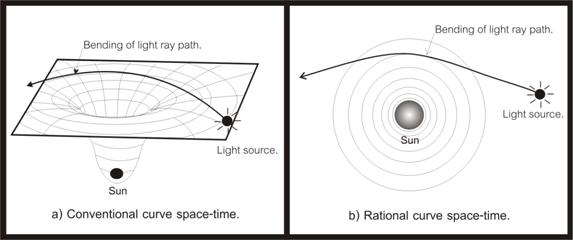Figure 11. Light ray path through curve space-time.

More problems about the conventional empty universe that could be solved using the new idea of Vacuum medium universe such as curvature of the universe, hypersphere of the universe, etc. Also we would found that it is easy to use the concept of vacuum medium space-time explain how gravity wave could be propagated via the vibration of vacuum medium space-time. And because it is the same mechanism as light wave, then the gravity wave speed is also equal to c!

7) Improving quantum mechanical theory. Now to improve QMT, we will use the same concept of vacuum medium space as was done for improving EMT, STR and GTR mentioned early. Armed with vacuum mechanics we could complete the mysterious conventional QMT to a rational and understandable one! Please see detail of this section in article CQMT , here is a short summary.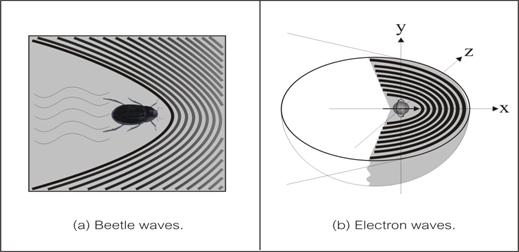Figure 12. Matter wave and particle wave.

Mechanism of particle wave.  It is easy to understand particle wave such as electron wave, by using method of analogy of the ōsurface water wavesö created by a moving water beetle as show in the diagram fig. 12 (a). When a beetle is moving, it will disturb surrounding water and creating a co-moving wave. In the same way the moving electron will disturb the surrounding vacuum medium and creating a co-moving wave-packet as show in the diagram fig. 12 (b). Note that the diagram shown is a three dimensions which was cut horizontally in x ¢ z plane to show only the lower half part.

In short, beetle wave was classified as linear dispersion of water surface wave, it involves with group velocity of capillary waves, while electron wave is a rotational transverse standing wave of vacuum medium. Anyway, there are two different points between them; first the beetle wave is a 2-dimension wave, while electron wave is a 3-dimension wave. Second, beetle wave is a vertical transverse of water surface wave, while electron wave is a rotational transverse wave-packet of vacuum medium!

Now, once we got a physical model of de Broglie (particle) waves, new derivation of de Broglie wave relation based on wave-packet of vacuum medium is possible. And then it leads us to do a more rational derivation of Schrödinger equation. So the old mysterious quantum mechanics was change to ōquantum vacuum mechanicsö, i.e. quantum mechanics of vacuum medium which is now could be understood by anyone!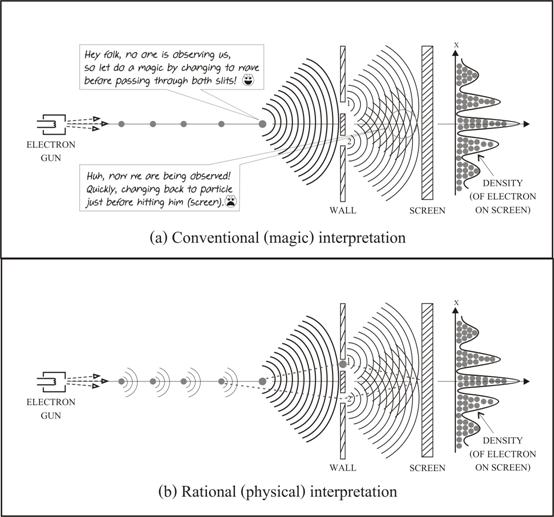Figure 13. Wave collapse interpretation.

Next, based on quantum vacuum mechanics, it is easy to explain the existing problems in the conventional theory. Indeed all the problems could be solved under the new concept, and here is the explanation of one of the most crazy problem; the well known ōwave collapse interpretationö!

According to conventional interpretation as shown in diagram fig. 13 (a), there is no explanation how each electron convert itself into waves, passing through both slits and then the two part of waves collapse back into a single particle on screen. It is something like the ōmagic of electrons! But, armed with the concept that particle wave is the co-moving wave of vacuum medium, it is easy to visualize and understand how electrons do the magic (as below).

In diagram fig. 13 (b), all the electron particles moving together with their own created (particle) waves. Some of electrons pass through slit 1, while others pass through slit 2 randomly. While each electron passes through slit 1 or 2, its co-moving waves will pass through both slit 1and 2 at the same time. After passing through both slits, both parts of the split waves will interfere each other while moving toward screen. Because the constructive interference zone carries most of the energy of waves (the high probability density), then it will guide the co-moving electron to follow within the zone!

Note, even through that the interference pattern which occurred on screen come from stream of electrons, but it is not the interference between two electrons; instead it comes from one single electron which interfere with itself! Of course, one electron can not create interference pattern, only a large amount of them could do. And this principle is also applied to photon, and which means that each photon will interfere with itself forming interference pattern!

8) Relativity and quantum theory could be married now! Here we will see how to solve the two main points of the inconsistency between GTR and QMT by using vacuum mechanics, i.e. the mechanism of vacuum medium as follow.

First, let us consider about the different amount of the existing of energy in vacuum space, between both theories. For GTR, vacuum space is an empty vacuum space without any energy, while QMT vacuum space is full with virtual particles Æsea, i.e. infinite vacuum energy. Armed with the new concept of vacuum medium space, we will show that both theories have the same amount of energy in vacuum space, i.e. vacuum medium energy!

Actually we have seen that vacuum medium energy which is formed to be the fabric structure of our universe is more rational than empty vacuum space or infinite vacuum energy space. The reason is because it serves as the physical mechanisms for both theories in the same one world! The only difference is that, while GTR was responsible for the macro world, QMT was responsible for the micro world. That is vacuum medium (energy) provides the gravity mechanism for GTR, and at the same time it also provides the mechanism for quantum phenomena in QMT such as particle wave.

Next, it was misunderstood that the concept of quantized energy in quantum mechanics is the same idea as something in classical mechanics such as the mechanical oscillation system of clock spring and pendulum. The reason comes from misinterpretation of Planck idea about the concept of atomic oscillator, which was thought that it is a normal continuous oscillator but generating quantized energy instead of a continuous energy. Actually, this atomic oscillator is not the same thing as a normal oscillator in classical mechanics; it is an impulse oscillator!

It is easy to visualize the difference between a short portion of wave train (wave-packet or discrete amount) and a continuous wave train which occurred in water wave. By applying a short duration of the disturbance source such as dropping a stone in water then wave-packet of water could be created, while a continuous wave train could be generated by dipping a continuous vibrating fork in water!

Analogously for electromagnetic waves, wave-packet of light (photon) is a short portion of wave train of electromagnetic wave, while radio wave which is man-made electromagnetic wave is a continuous wave. The reason is because radio wave was created from a continuous varying current source (please see detail in CEMT ).

By the way, normally when talking about an oscillator both in classical mechanics or electromagnetic oscillating system, we usually mean a continuous oscillator. And in our daily experience we could see both types of oscillator as mentioned in the early paragraph, but thing is different in atomic ream in which only the impulse oscillator is possible!

In conclusion, because of our ignorance about mechanism of PlanckÆs radiation energy, so we think that the concept of quantized energy is also true for classical mechanics. Now based on vacuum mechanics concept, then we found that actually it was limited within quantum ream and not for the classical one. So we can conclude that there is no contradiction about the quantity of energy between GTR and QMT!

9) Appearance of the theory of everything. Up to now we have seen some clues of TOE, i.e. the unifying of the four fundamental forces of the nature; gravitational force, electromagnetic force, weak force and strong force. Here is the summary.

Based on the new concept of vacuum medium space, i.e. the vacuum space was created from vacuum medium.  And the intrinsic property of vacuum medium is that each of its infinisimal part attracts each other by its own contraction force and forming to be the fabric structure of our universe. So we could say that gravity is the property of vacuum medium. Together with the concept that electron and proton are tiny black holes of condensed vacuum medium, which forming to be the basic unit of matter, i.e. hydrogen atom and helium atom. And because matter is composed of billion of atoms, then gravity is also the property of matter!

From the concept that electron and proton are tiny black holes of condensed vacuum medium, it is not surprise that they must attract each other. This attractive force is the appearance of the familiar force i.e. electromagnetic force (Coulomb electric force)! Anyway, there is a more complicate situation about this force i.e. both attraction force and repulsion force are involved. But this situation could be explained that it arises from the net result force between black hole force and internal stress of the vacuum medium in between. So this will change the conventional concept of ōdifferent type of charge particles attract while the same types repelö to a better understandable concept of ōdifferent size of charge particles attract while the same sizes repelö!

The next, is the third force, i.e. weak force which is the attach force occurred between proton and electron in a neutron. Actually a proton and an electron attract each other in a hydrogen atom, but if it was forced to attach to each other then a neutron is formed. So the additional force used to overcome the internal stress of the vacuum medium in between, and make it possible to attach together is the mentioned weak force!

The last, is the fourth force i.e. strong force which is the attach force between two protons and two neutrons in helium atom. Normally two protons attract each other when they close together for a certain distance because they are black holes. But when they are closer more than the previous mentioned distance then they will repel each other because of the internal stress of the vacuum medium in between. So to make them attach together, an external force was required, and the mentioned force is strong force!

Finally, we could notice that all the four forces are the consequence of the mechanism of vacuum medium, i.e. vacuum mechanics. So we could say that we have unified the four basic forces of nature! Anyway, some one may doubt why TOE which unifies the four forces was called as the theory of everything, why it is not include matter or how matter is connected with the four forces? The answer may be because physicists think that matter comes from force or matter is force. Armed with vacuum mechanics we would found that the answer is obvious, i.e. matter is a bulge of condensed vacuum medium, so it is also the same one of the mechanism of vacuum medium. This should be the complete theory of everything!

10) Conclusion & discussion. Up to now, we have learnt how to improve the four main conventional modern physics theories i.e. EMT, STR, GTR and QMT by adding the new concept of vacuum mechanics (i.e. the mechanism of vacuum medium). We have shown how suitable and understandable models were built, what their advantages are, how they could solve the existing problems and finally pave the way to TOE! Here are some additional comments;

a) Actually, what we have done is just ōput vacuum mechanics into physics theoriesö. We give the mechanism of vacuum medium for electromagnetic phenomena in CEMT, we show that relativistic effects was due to the mechanism of vacuum medium in CSTR, we change the magic gravity to a physical one by adding the mechanism of vacuum medium in CGTR, and finally we turn the ghost field of particle wave to a realistic wave of vacuum medium in CQMT. All of these natural phenomena are the appearances of the mechanism of vacuum medium, i.e. vacuum mechanics ¢ A new approach to the theory of everything!

b) Nowadays, the hottest issue in scientific community is about Large Hadrons Collider Project for finding Higgs particle by using standard model of particle physics theory. Anyway, for someone who familiar with the theory would found that the theory weak point is not different from the four main theories we talked about, but even worse! Beside its successful in prediction about any (non-gravitational) process in the universe, the standard model was criticized as an extraordinary ad hoc and ugly theory that it was clearly nonsense.

For the author opinion, we could also improve the theory by using our new concept vacuum mechanics in the same way as we have done with the four main theories. What we will get is a complete theory which could explain how it works beside its successful in prediction!

c) Let us talk a little about Higgs particle which was praised as god particle! And this particle was told to be created from the condensed of Higgs field, in which it was designed to be something that give mass to all the existing particles via the process called Higgs mechanism.

Anyway, the problem is that we do not know what the Higgs field is; it was told that it is something like the old aether which pervade in vacuum space. Armed with the concept of vacuum medium space, it is not difficult to see that actually the mentioned Higgs field is the same thing as our vacuum medium

d) Lastly, we could see that what was written in this paper is a short one, and it is not fully mathematical rigor. What we have done is just enough to give the new idea to the readers with some confidences. So what was left here should be the work of people who are involved in the matter to complete it for the virtue of academic merit and the progression of our worldÆs knowledge!

11) References.

(Precaution; Several text books with different authors were used as the reference and each author used different pattern and different notation for the same formula. Here in this paper, the author has made some change of the original pattern and notation for the readers convenient, so please be careful!)

 D. Halliday, R. Resnick, and K. Krane, ōPhysicsö Vol. 2 Extended Version Fourth Edition, p. 1191-1192.

 Stephen Hawking, ōHawking muses on ultimate theory of the universeö in MIT News, January 23 2003.

 James Blake Westgard, ōElectrodynamics: A Concise Introductionö, p.87-95.

 Constantine A. Balanis ōAntenna Theory, Analysis and Designö, p.7-11.

 Oleg D. Jefimenko, ōCausality Electromagnetic Induction and Gravitationö Second Edition, p. 13-16.

 Richard P. Feynman, ōThe Character of Physical Lawö, p.129. For [6Æ] p.145.

 Tian Yu Cua, ōConceptual Development of 20Pth Century Field Theoriesö, p. 10-14.

 Arnold Sommerfeld, ōMechanics of Deformable Bodiesö, p. 107. For [8Æ] p.108 ¢ 111.

 John D. Ryder, ōNetwork, Lines and Fieldsö Second Edition, p. 553-555.

 Editors: Richard C Johnson, Henry Jasik, ōAntenna Applications Reference Guideö, p. 2-13 to 2-15.

 W. Pauli, ōTheory of Relativityö, p. 202-205.

 Ray dÆInverno ōIntroducing EinsteinÆs Relativityö Reprinted 1993 p. 239-243.

 Richard P. Feynman, Robert B. Leightton and M. Sands, ōThe Feynman Lectures on Physicsö Vol. 2,

p.28-1 to 28-4.

 Sir Horace Lamb, ōHydrodynamicsö, p. 124.

 Nimit Theeraleekul, ōöCompleted Maxwell electromagnetic field theory (CEMT)ö, now presenting in

 Nimit Theeraleekul, ōöCompleted Einstein special theory of relativity (CSTR)ö, now presenting in

 Nimit Theeraleekul, ōöCompleted Einstein general theory of relativity (CGTR)ö, now presenting in

 Nimit Theeraleekul, ōöCompleted quantum mechanical theoryö (CQMT), now presenting in

ģģģģģģģģģģģ.